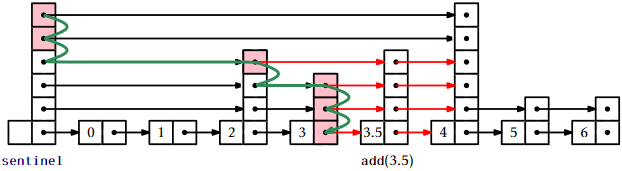# 4.2: SkiplistSSet - An Efficient SSet

$$\newcommand{\vecs}{\overset { \rightharpoonup} {\mathbf{#1}} }$$ $$\newcommand{\vecd}{\overset{-\!-\!\rightharpoonup}{\vphantom{a}\smash {#1}}}$$$$\newcommand{\id}{\mathrm{id}}$$ $$\newcommand{\Span}{\mathrm{span}}$$ $$\newcommand{\kernel}{\mathrm{null}\,}$$ $$\newcommand{\range}{\mathrm{range}\,}$$ $$\newcommand{\RealPart}{\mathrm{Re}}$$ $$\newcommand{\ImaginaryPart}{\mathrm{Im}}$$ $$\newcommand{\Argument}{\mathrm{Arg}}$$ $$\newcommand{\norm}{\| #1 \|}$$ $$\newcommand{\inner}{\langle #1, #2 \rangle}$$ $$\newcommand{\Span}{\mathrm{span}}$$ $$\newcommand{\id}{\mathrm{id}}$$ $$\newcommand{\Span}{\mathrm{span}}$$ $$\newcommand{\kernel}{\mathrm{null}\,}$$ $$\newcommand{\range}{\mathrm{range}\,}$$ $$\newcommand{\RealPart}{\mathrm{Re}}$$ $$\newcommand{\ImaginaryPart}{\mathrm{Im}}$$ $$\newcommand{\Argument}{\mathrm{Arg}}$$ $$\newcommand{\norm}{\| #1 \|}$$ $$\newcommand{\inner}{\langle #1, #2 \rangle}$$ $$\newcommand{\Span}{\mathrm{span}}$$$$\newcommand{\AA}{\unicode[.8,0]{x212B}}$$

A SkiplistSSet uses a skiplist structure to implement the SSet interface. When used in this way, the list $$L_0$$ stores the elements of the SSet in sorted order. The $$\mathtt{find(x)}$$ method works by following the search path for the smallest value $$\mathtt{y}$$ such that $$\mathtt{y}\ge\mathtt{x}$$:

    Node<T> findPredNode(T x) {
Node<T> u = sentinel;
int r = h;
while (r >= 0) {
while (u.next[r] != null && compare(u.next[r].x,x) < 0)
u = u.next[r];   // go right in list r
r--;               // go down into list r-1
}
return u;
}
T find(T x) {
Node<T> u = findPredNode(x);
return u.next == null ? null : u.next.x;
}


Following the search path for $$\mathtt{y}$$ is easy: when situated at some node, $$\mathtt{u}$$, in $$L_{\mathtt{r}}$$, we look right to $$\texttt{u.next[r].x}$$. If $$\mathtt{x}>\texttt{u.next[r].x}$$, then we take a step to the right in $$L_{\mathtt{r}}$$; otherwise, we move down into $$L_{\mathtt{r}-1}$$. Each step (right or down) in this search takes only constant time; thus, by Lemma 4.1.1, the expected running time of $$\mathtt{find(x)}$$ is $$O(\log \mathtt{n})$$.

Before we can add an element to a SkipListSSet, we need a method to simulate tossing coins to determine the height, $$\mathtt{k}$$, of a new node. We do so by picking a random integer, $$\mathtt{z}$$, and counting the number of trailing $$1$$s in the binary representation of $$\mathtt{z}$$:1

    int pickHeight() {
int z = rand.nextInt();
int k = 0;
int m = 1;
while ((z & m) != 0) {
k++;
m <<= 1;
}
return k;
}


To implement the $$\mathtt{add(x)}$$ method in a SkiplistSSet we search for $$\mathtt{x}$$ and then splice $$\mathtt{x}$$ into a few lists $$L_0$$,..., $$L_{\mathtt{k}}$$, where $$\mathtt{k}$$ is selected using the $$\mathtt{pickHeight()}$$ method. The easiest way to do this is to use an array, $$\mathtt{stack}$$, that keeps track of the nodes at which the search path goes down from some list $$L_{\mathtt{r}}$$ into $$L_{\mathtt{r}-1}$$. More precisely, $$\mathtt{stack[r]}$$ is the node in $$L_{\mathtt{r}}$$ where the search path proceeded down into $$L_{\mathtt{r}-1}$$. The nodes that we modify to insert $$\mathtt{x}$$ are precisely the nodes $$\mathtt{stack},\ldots,\mathtt{stack[k]}$$. The following code implements this algorithm for $$\mathtt{add(x)}$$:

    boolean add(T x) {
Node<T> u = sentinel;
int r = h;
int comp = 0;
while (r >= 0) {
while (u.next[r] != null
&& (comp = compare(u.next[r].x,x)) < 0)
u = u.next[r];
if (u.next[r] != null && comp == 0) return false;
stack[r--] = u;          // going down, store u
}
Node<T> w = new Node<T>(x, pickHeight());
while (h < w.height())
stack[++h] = sentinel;   // height increased
for (int i = 0; i < w.next.length; i++) {
w.next[i] = stack[i].next[i];
stack[i].next[i] = w;
}
n++;
return true;
}Figure $$\PageIndex{1}$$: Adding the node containing $$3.5$$ to a skiplist. The nodes stored in $$\mathtt{stack}$$ are highlighted.

Removing an element, $$\mathtt{x}$$, is done in a similar way, except that there is no need for $$\mathtt{stack}$$ to keep track of the search path. The removal can be done as we are following the search path. We search for $$\mathtt{x}$$ and each time the search moves downward from a node $$\mathtt{u}$$, we check if $$\texttt{u.next.x}=\mathtt{x}$$ and if so, we splice $$\mathtt{u}$$ out of the list:

    boolean remove(T x) {
boolean removed = false;
Node<T> u = sentinel;
int r = h;
int comp = 0;
while (r >= 0) {
while (u.next[r] != null
&& (comp = compare(u.next[r].x, x)) < 0) {
u = u.next[r];
}
if (u.next[r] != null && comp == 0) {
removed = true;
u.next[r] = u.next[r].next[r];
if (u == sentinel && u.next[r] == null)
h--;  // height has gone down
}
r--;
}
if (removed) n--;
return removed;
}Figure $$\PageIndex{2}$$: Removing the node containing $$3$$ from a skiplist.

## $$\PageIndex{1}$$ Summary

The following theorem summarizes the performance of skiplists when used to implement sorted sets:

Theorem $$\PageIndex{1}$$.

SkiplistSSet implements the SSet interface. A SkiplistSSet supports the operations $$\mathtt{add(x)}$$, $$\mathtt{remove(x)}$$, and $$\mathtt{find(x)}$$ in $$O(\log \mathtt{n})$$ expected time per operation.

#### Footnotes

1This method does not exactly replicate the coin-tossing experiment since the value of $$\mathtt{k}$$ will always be less than the number of bits in an $$\mathtt{int}$$. However, this will have negligible impact unless the number of elements in the structure is much greater than $$2^{32}=4294967296$$.

This page titled 4.2: SkiplistSSet - An Efficient SSet is shared under a CC BY license and was authored, remixed, and/or curated by Pat Morin (Athabasca University Press) .